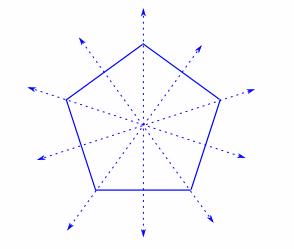Courses

# Test: Where To Look From - 1

## 10 Questions MCQ Test Mathematics (Maths) for Class 3 | Test: Where To Look From - 1

Description
This mock test of Test: Where To Look From - 1 for Class 3 helps you for every Class 3 entrance exam. This contains 10 Multiple Choice Questions for Class 3 Test: Where To Look From - 1 (mcq) to study with solutions a complete question bank. The solved questions answers in this Test: Where To Look From - 1 quiz give you a good mix of easy questions and tough questions. Class 3 students definitely take this Test: Where To Look From - 1 exercise for a better result in the exam. You can find other Test: Where To Look From - 1 extra questions, long questions & short questions for Class 3 on EduRev as well by searching above.
QUESTION: 1

### How many lines of symmetry does this Octagon have?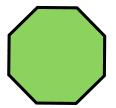Solution: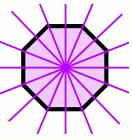QUESTION: 2

### John notices that a standard piece of paper is a rectangle. How many lines of symmetry does it have?

Solution: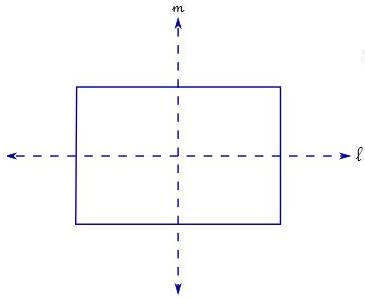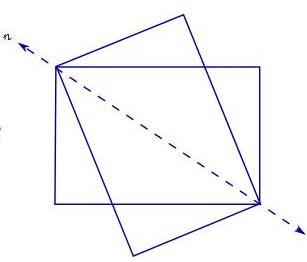It has just 2 lines of symmetry, l and m
Some people think that the lines along the diagonals (like n) are also lines of symmetry; but when you reflect along that line n, the image does not fit the outline of the original.

QUESTION: 3

### How many lines of symmetry does this star have?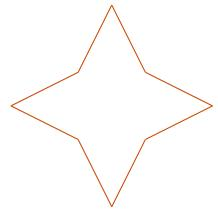Solution: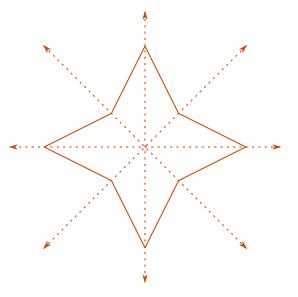It has 4 lines of symmetry

QUESTION: 4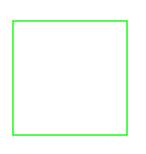How many lines of symmetry does the square have?

Solution:

It has four lines of symmetry: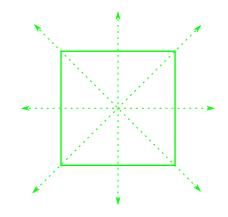QUESTION: 5

Jo wants this image to have an axis of symmetry: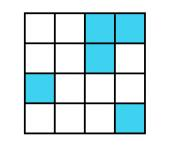What is the smallest number of the little squares that Jo has to shade in to get at least one axis of symmetry?

Solution:

Two squares must be shaded. The following diagram shows the two squares and the axis of symmetry: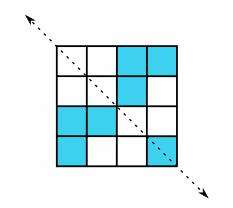QUESTION: 6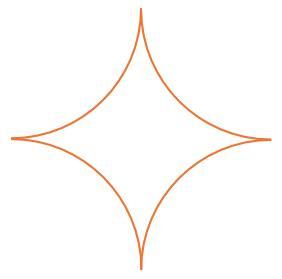The diagram shows a shape made up of four quarter-circular arcs joined together. How many lines of symmetry does it have?

Solution: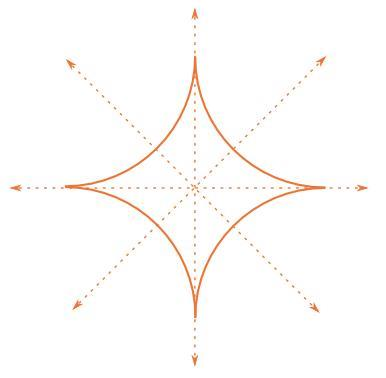The diagram shows that it has 4 lines of symmetry.

QUESTION: 7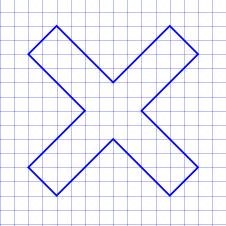How many lines of symmetry does this letter X have?

Solution: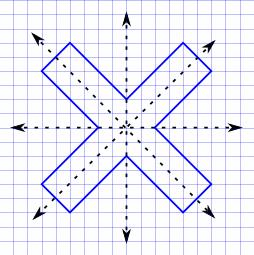It has 4 lines of symmetry.

QUESTION: 8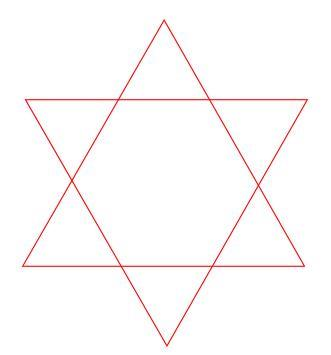How many lines of symmetry does the Star of David have?

Solution:The diagram shows that it has 6 lines of symmetry.

QUESTION: 9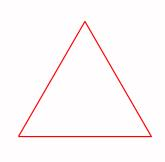How many lines of symmetry does the equilateral triangle have?

Solution:

It has three lines of symmetry: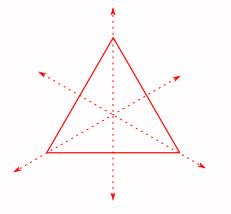QUESTION: 10

A 5-sided figure with all its sides equal and all its angles equal is called a regular pentagon: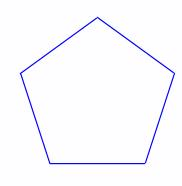How many lines of symmetry does the regular pentagon have?

Solution:

It has five lines of symmetry: